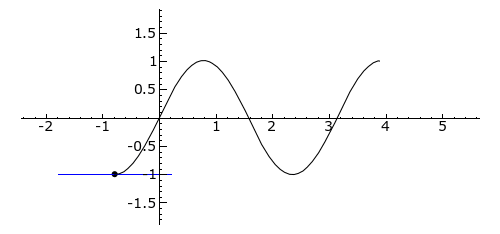# Gamma and Delta of a Stock

I cant visualize these two concepts.

Why do we say that the gamma of a stock is 0 and why do we say that a stock has a delta of 1?

Try delta first. Delta is the change in the price of a security (e.g., an option) divided by the change of the price of the underlying. (If you know differential calculus, you’ll understand that delta is the first derivative of the price of the security with respect to the price of the underlying.)

So, when the price of the stock changes by, say, USD 0.50, by how much does the price of the stock change? What’s the ratio?

1 Like

In addition to @S2000magician’s answer, you can visualize by looking at the graph below that Delta is the first derivative and Gamma is the second derivative (the derivative of the derivative of a function).its 0.5/0.5 which is =1 and the gamma being the second derivative of delta and 1 being a constant, gamma is 0

Bingo!

(Well . . . almost Bingo! Gamma is the second derivative of price with respect to price of the underlying, but it’s the (first) derivative of delta with respect to the price of the underlying.)

1 Like

thanks magician

I do have two more questions.

Based on the put call parity, the gamma of a call = gamma of a put call and the vega of a call =the vega of a put.
Put call parity formula: S+P= C+PV(X)
So I understood that gamma of a stock is equal to 0 which leaves us with the put option on the left side, but what about the present value of the exercise price . What happens to it? How do we arrive to the conclusion of that the gamma of a put =gamma of a call? and vega of a put=vega of a call?

When the price of the stock changes, what happens to the present value of the strike price?

When the volatility of the stock’s returns changes, what happens to the present value of the strike price?

it doesnt change

So . . . the delta, gamma, and vega of the bond’s price are . . . ?

unrelated to the stock price?

Yes.

And their values are . . . ?